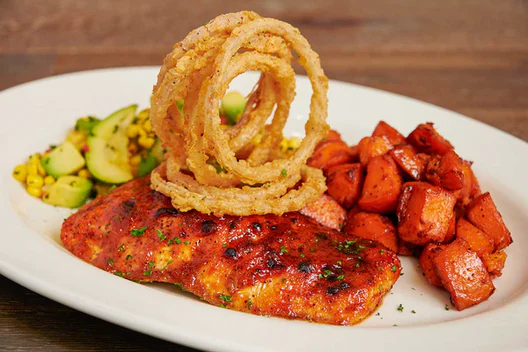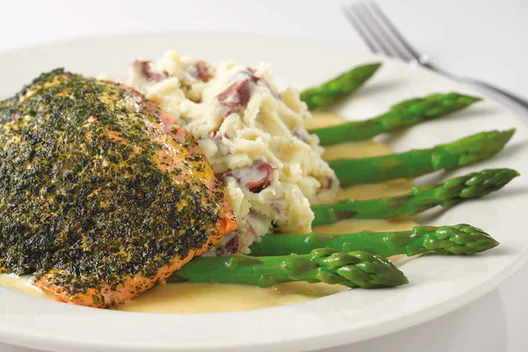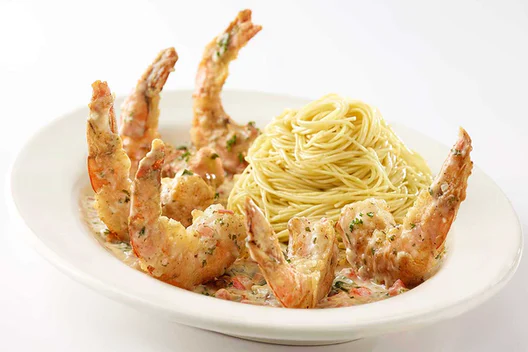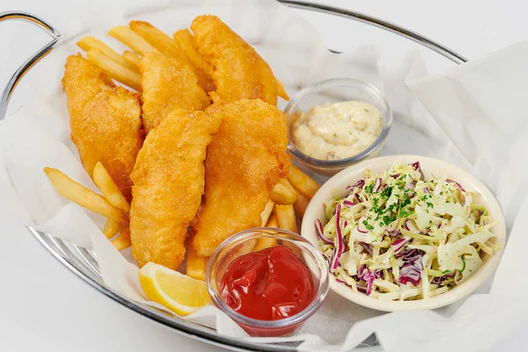What is the healthiest pescatarian option from The Cheesecake Factory?

Hopefully this helps any other pescatarians trying to decide which dinner menu selection they want if focusing on healthy selections. ^_^

** All images are from The Cheese Cake Factory's website.1. Fresh Grilled Salmon
Calories = 1240
Total Fat (g) = 720
Fat Calories = 80
Saturated Fat (g) = 26
Trans Fat (g) = 1
Cholesterol (mg) = 225
Sodium (mg) = 1740
Total Carbs (g) = 66
Fiber (g) = 10
Sugars (g) = 6
Protein (g) = 632. Jamaican Black Pepper Shrimp
Calories = 1260
Total Fat (g) = 250
Fat Calories = 27
Saturated Fat (g) = 7
Trans Fat (g) = 0
Cholesterol (mg) = 360
Sodium (mg) = 1990
Total Carbs (g) = 186
Fiber (g) = 16
Sugars (g) = 60
Protein (g) = 673. Miso Salmon
Calories = 1340
Total Fat (g) = 610
Fat Calories = 67
Saturated Fat (g) = 31
Trans Fat (g) = 1.5
Cholesterol (mg) = 240
Sodium (mg) = 1430
Total Carbs (g) = 120
Fiber (g) = 5
Sugars (g) = 27
Protein (g) = 644. Carolina Grilled Salmon
Calories = 1360
Total Fat (g) = 660
Fat Calories = 74
Saturated Fat (g) = 19
Trans Fat (g) = 1
Cholesterol (mg) = 190
Sodium (mg) = 2200
Total Carbs (g) = 113
Fiber (g) = 15
Sugars (g) = 42
Protein (g) = 635. Herb Crusted Filet of Salmon
Calories = 1350
Total Fat (g) = 830
Fat Calories = 93
Saturated Fat (g) = 43
Trans Fat (g) = 2.5
Cholesterol (mg) = 290
Sodium (mg) = 1620
Total Carbs (g) = 65
Fiber (g) = 5
Sugars (g) = 4
Protein (g) = 646. Shrimp Scampi
Calories = 1350
Total Fat (g) = 690
Fat Calories = 77
Saturated Fat (g) = 37
Trans Fat (g) = 2
Cholesterol (mg) = 335
Sodium (mg) = 2800
Total Carbs (g) = 123
Fiber (g) = 8
Sugars (g) = 7
Protein (g) = 457. Fish and Chips
Calories = 1860
Total Fat (g) = 1090
Fat Calories = 121
Saturated Fat (g) = 24
Trans Fat (g) = 1
Cholesterol (mg) = 195
Sodium (mg) = 2940
Total Carbs (g) = 133
Fiber (g) = 8
Sugars (g) = 30
Protein (g) = 538. Fried Shrimp Platter
Calories = 1920
Total Fat (g) = 940
Fat Calories = 104
Saturated Fat (g) = 21
Trans Fat (g) = 1
Cholesterol (mg) = 270
Sodium (mg) = 3140
Total Carbs (g) = 191
Fiber (g) = 13
Sugars (g) = 38
Protein (g) = 50